# Simple probability

##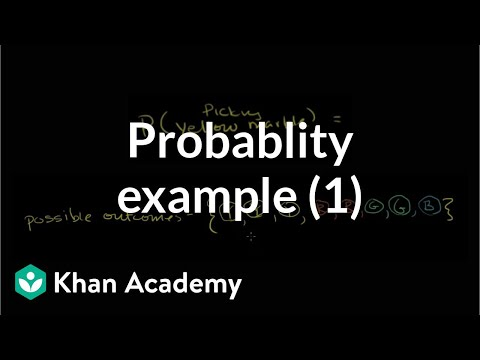By Khan Academy

In order to find the probability of picking a yellow marble from a bag, we have to first determine the number of possible outcomes and how then many of them meet our constraints.# Simple probability

##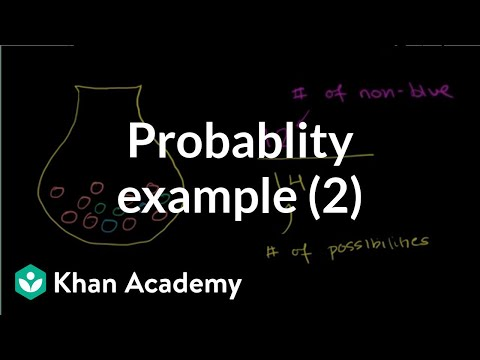By Khan Academy

In this example we are figuring out the probability of randomly picking a non-blue marble from a bag. Again, we'll have to think about the possible outcomes first.# Simple probability

##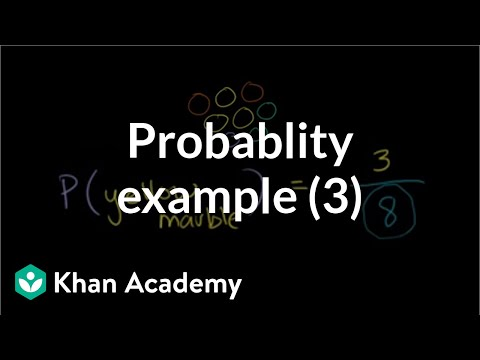By Khan Academy

Now we're working out the probability of randomly selecting a yellow marble from a bag of multi-colored marbles. Let's practice together.# Small Sample Hypothesis Test

##By Khan Academy

This video lecture series on Introduction to Statistics includes Average Sample vs Population Variance Standard Deviation Random Variables Probability density functions Binomial distribution Poisson Process Law of Large numbers and Normal Distribution......# Probability: Finding What Percent an Event Has of Occurring

##By Khan Academy

The instructor in this video Sal Khan discusses how to find the probability that an event will occur. He uses an electronic chalkboard to demonstrate several examples using a step by step approach.# Probability models

##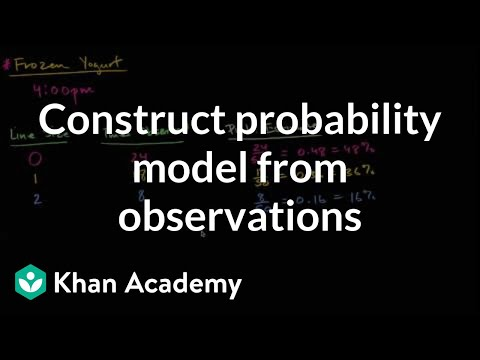By Khan Academy

Model the probability of a frozen yogurt line having 0, 1, or 2 people in it.# Khan Academy- Classifying Events as Independent or Dependent

##By Khan Academy

Have you ever taken a test and discovered you have no choice but to guess on a couple of problems? In this example problem we are considering the probability of two independent events occurring.# Example: Bag of unfair coins

##By Khan Academy

Example where the probability of an outcome is dependent on which coin you happen to pick# Comparing probabilities

##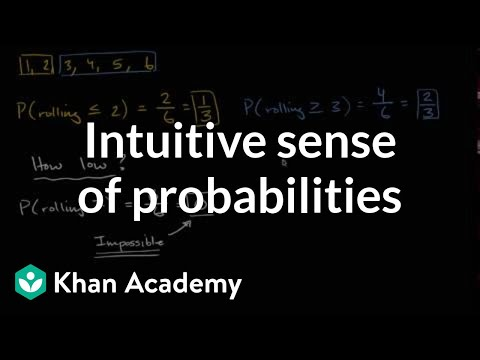By Khan Academy

Think about what probabilities really mean. What does a probability of 0 mean? How about 1?# Calculating Probability of Multiple Events

##By The Free Math Tutor

This video illustrates how to calculate the Probability of Multiple Events. For part 2 of this video including examples 3 4 and 5 as well as many more instructional Math videos and exercise and answer sheets go to: http://www.thefreemathtutor.com# Introduction to Random Variables - Khan Academy

##By Khan Academy

This video lecture series from Khan Academy on Probability includes Introduction to Probability Permutations Combinations Probability using Combinations Conditional probability Introduction to Random Variables Probability Density Functions Binomial Distribution Expected Value Expected value of Binomial Distribution Poisson Process Law of Large Numbers.....# Khan Academy: Sampling Distribution of the Sample Mean

##By Khan Academy

This video lecture series on Introduction to Statistics includes Average Sample vs Population Variance Standard Deviation Random Variables Probability density functions Binomial distribution Poisson Process Law of Large numbers and Normal Distribution......# Reading Pie Graphs (Circle Graphs) - Khan Academy

##By Khan Academy

While pie charts (or circle graphs) are often easy to read they are more difficult to put into percentages since that usually involves fractions. When used to initially teach fractions this process usually is a bit easier. Use the easy to understand video in this learning to explain to students how to read pie charts (circle graphs).# Example: Probability of rolling doubles

##By Khan Academy

We're thinking about the probability of rolling doubles on a pair of dice. Let's create a grid of all possible outcomes.# Probability (Part 7)-More on Conditional Probability

##By Khan Academy

The instructor in this video Sal Khan continues his discussion of conditional probability while touching on Bayes' Theorem. Mr. Khan uses the Paint Program (with different colors) to illustrate his points. Sal Khan is the recipient of the 2009 Microsoft Tech Award in Education.# Probability -Probability of a Monopoly Roll - Khan Academy

##By Khan Academy

What's the probability of getting a certain roll in a boardgame with two dice? Fast forward to 6:47 to find out how to solve these types of questions without a grid.# Probability and Equally Likely Events

##By Art of Problem Solving

YouTube presents Probability and Equally Likely Events an educational video resource on math.# Probability -Flipping a Coin (Part 2)

##By Khan Academy

The probability of getting 7 tails in a row is 1/128. How come? We find the probability for EACH tails first. Any particular outcome is 1 out of 128 of the total number of outcomes. What's the probability of getting EXACTLY one heads? Watch the instructor explain starting at 2:20.# Probability -Flipping a Coin (Part 1)

##By Khan Academy

You may have seen the first part of this video on the previous learnboard for common core standard 7.SP.5. If not no worries. :-) This video reviews the probability in a series of coin tosses. Remember that EACH toss is independent so we must multiply each individual probability to find the total.